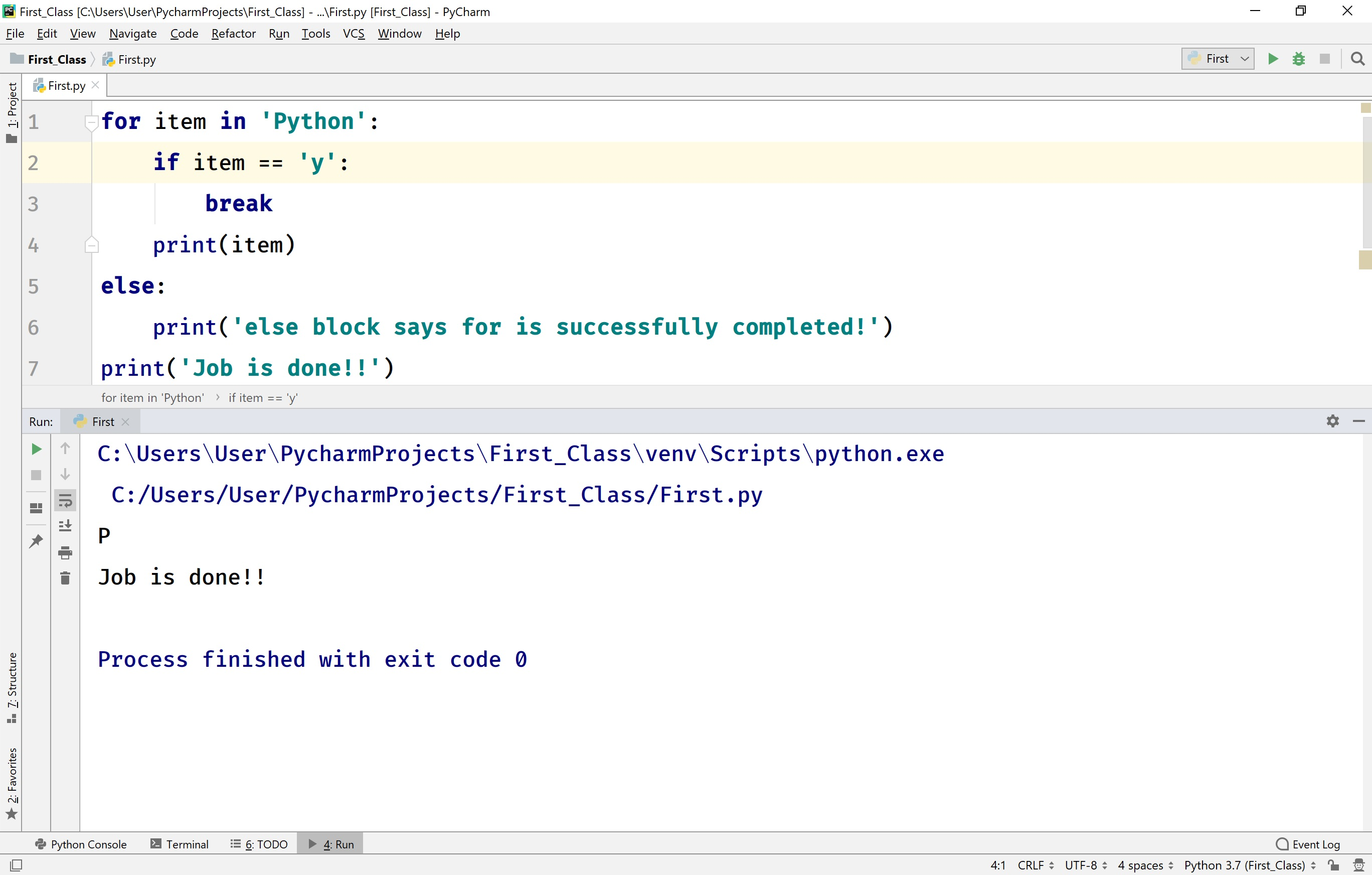The perfect place for easy learning...

×

# Python Looping Statements

In Python, the iterative statements are also known as looping statements or repetitive statements. The iterative statements are used to execute a part of the program repeatedly as long as a given condition is True. Python provides the following iterative statements.

• while statement
• for statement

## while statement

In Python, the while statement is used to execute a set of statements repeatedly. In Python, the while statement is also known as entry control loop statement because in the case of the while statement, first, the given condition is verified then the execution of statements is determined based on the condition result. The general syntax of while statement in Python is as follows.

### Syntax

```while condition:
Statement_1
Statement_2
Statement_3
...```

The execution flow of while statement is as shown in the following figure.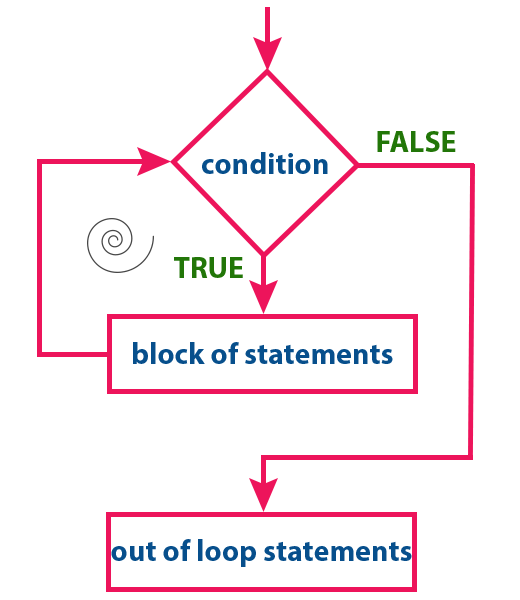When we define a while statement, the block of statements must be specified using indentation only. The indentation is a series of white-spaces. Here, the number of white-spaces may variable, but all statements must use the identical number of white-spaces. Let's look at the following example Python code.

# Python code to illustrate while statement

``````
count = int(input('How many times you want to say "Hello": '))
i = 1
while i <= count:
print('Hello')
i += 1
print('Job is done! Thank you!!')
```
```

When we run the above code, it produces the output as follows.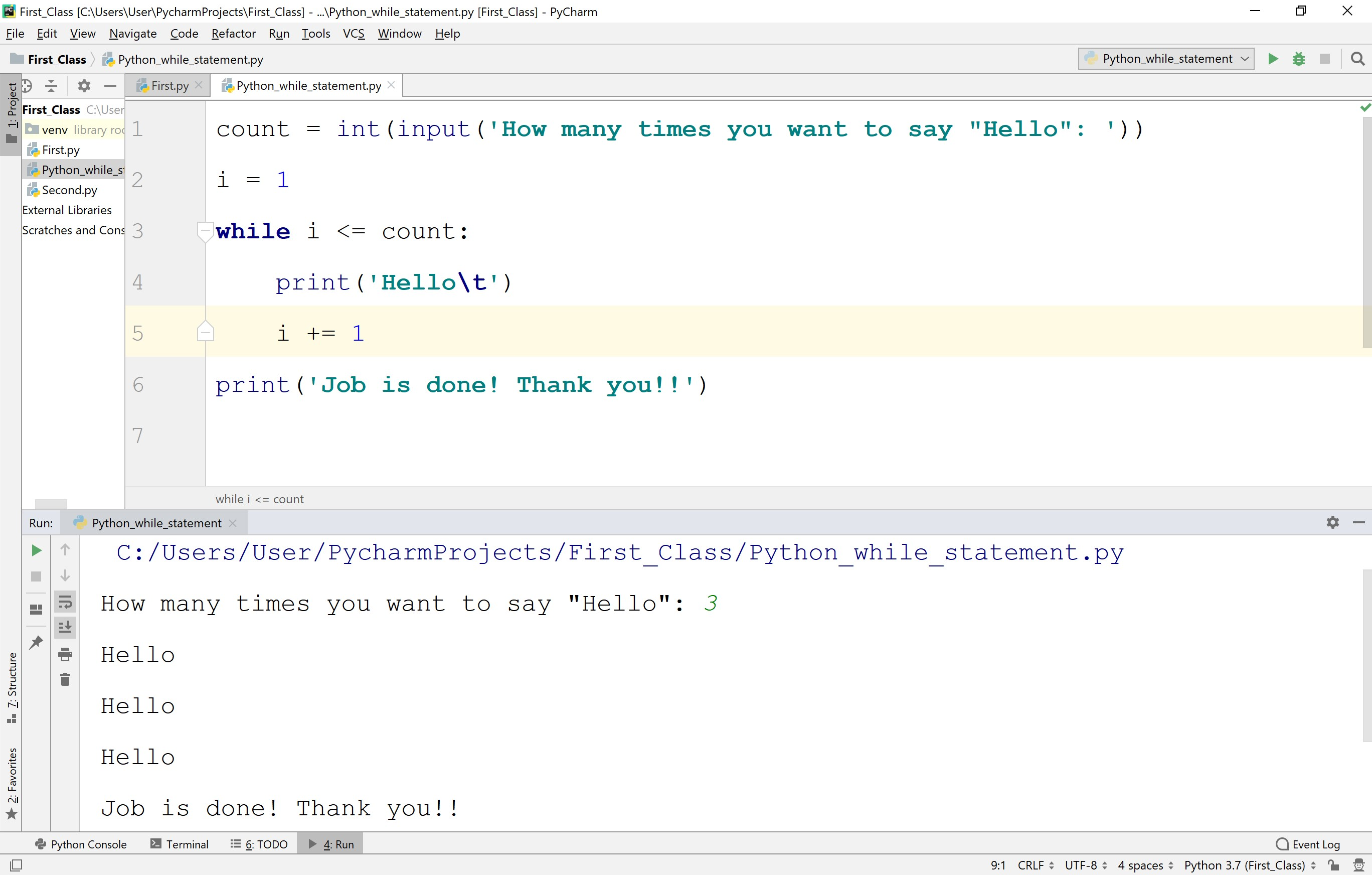• When we use a while statement, the value of the variable used in the condition must be modified otherwise while loop gets into the infinite loop.

## while statement with 'else' clause in Python

In Python, the else clause can be used with a while statement. The else block is gets executed whenever the condition of the while statement is evaluated to false. But, if the while loop is terminated with break statement then else doesn't execute.

# Python code to illustrate for statement with else clause

``````
# Here, else block is gets executed because break statement does not executed
count = int(input('How many times you want to say "Hello": '))
i = 1
while i <= count:
if count > 10:
print('I cann\'t say more than 10 times!')
break
print('Hello')
i += 1
else:
print('This is else block of while!!!')
print('Job is done! Thank you!!')
```
```

When we run the above code with count value 2, the else block is gets executed because a break statement does not execute. It produces the output as follows.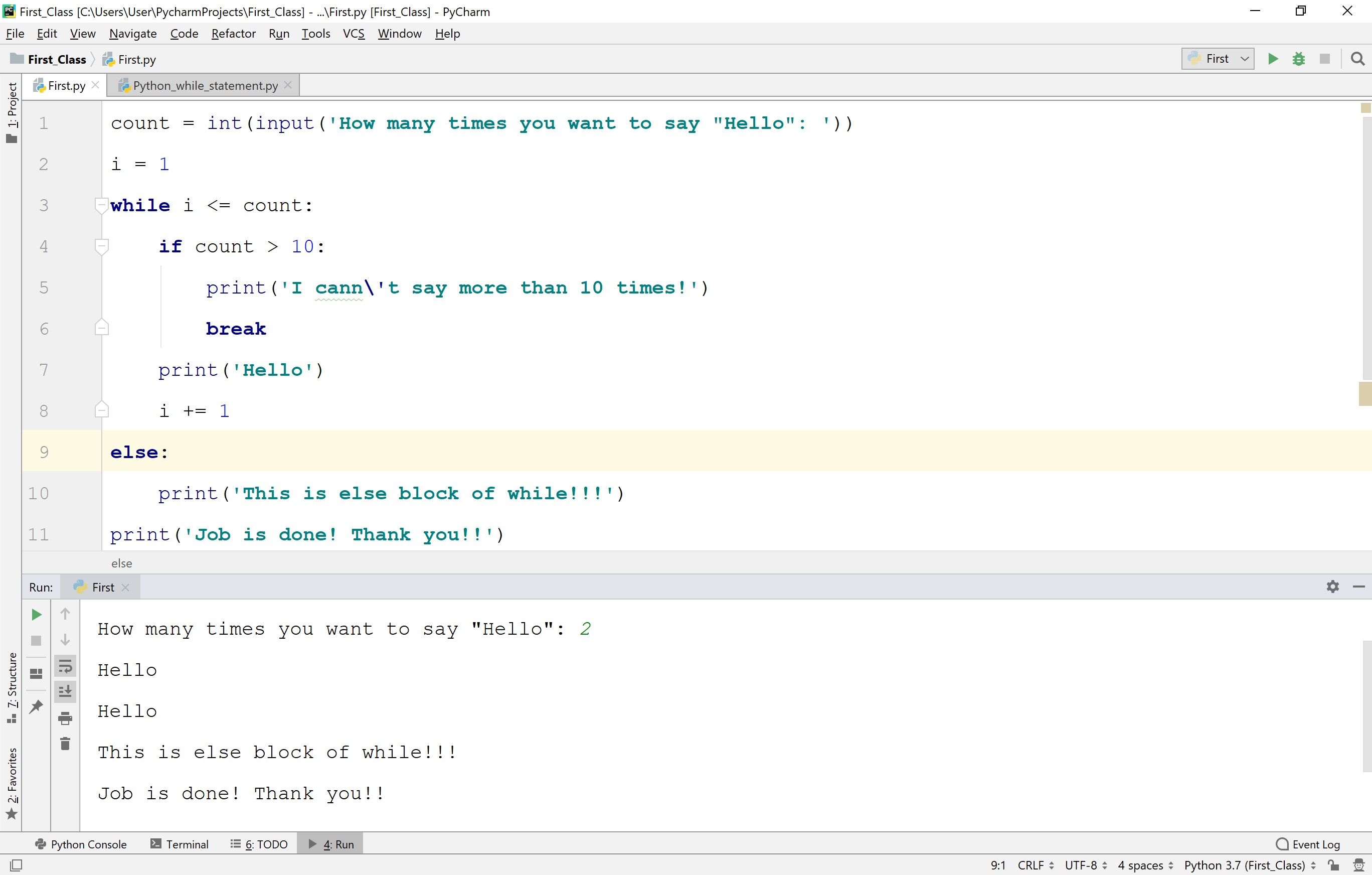When we run the above code with count value 12, the else block is not gets executed because the while loop is terminated with a break statement. It produces the output as follows.## for statement in Python

In Python, the for statement is used to iterate through a sequence like a list, a tuple, a set, a dictionary, or a string. The for statement is used to repeat the execution of a set of statements for every element of a sequence.

The general syntax of for statement in Python is as follows.

### Syntax

```for <variable> in <sequence>:
Statement_1
Statement_2
Statement_3
...```

In the above syntax, the variable is stored with each element from the sequence for every iteration.

# Python code to illustrate for statement with List

``````
# Python code to illustrate for statement with List
my_list = [1, 2, 3, 4, 5]
for value in my_list:
print(value)
print('Job is done!')
```
```

When we run the above code, it produces the output as follows.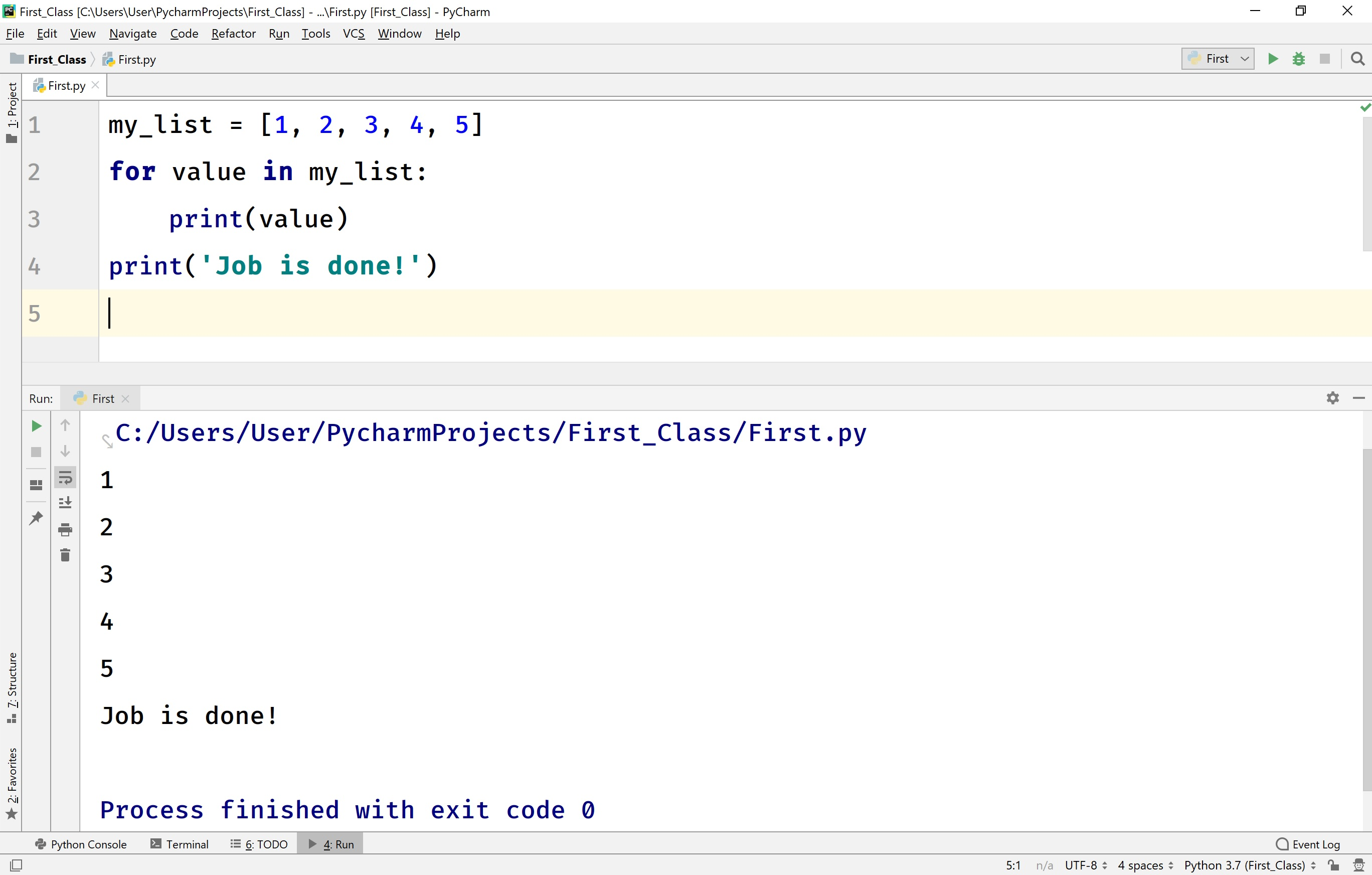# Python code to illustrate for statement with Tuple

``````
# Python code to illustrate for statement with Tuple
my_tuple = (1, 2, 3, 4, 5)
for value in my_tuple:
print(value)
print('Job is done!')
```
```

When we run the above code, it produces the output as follows.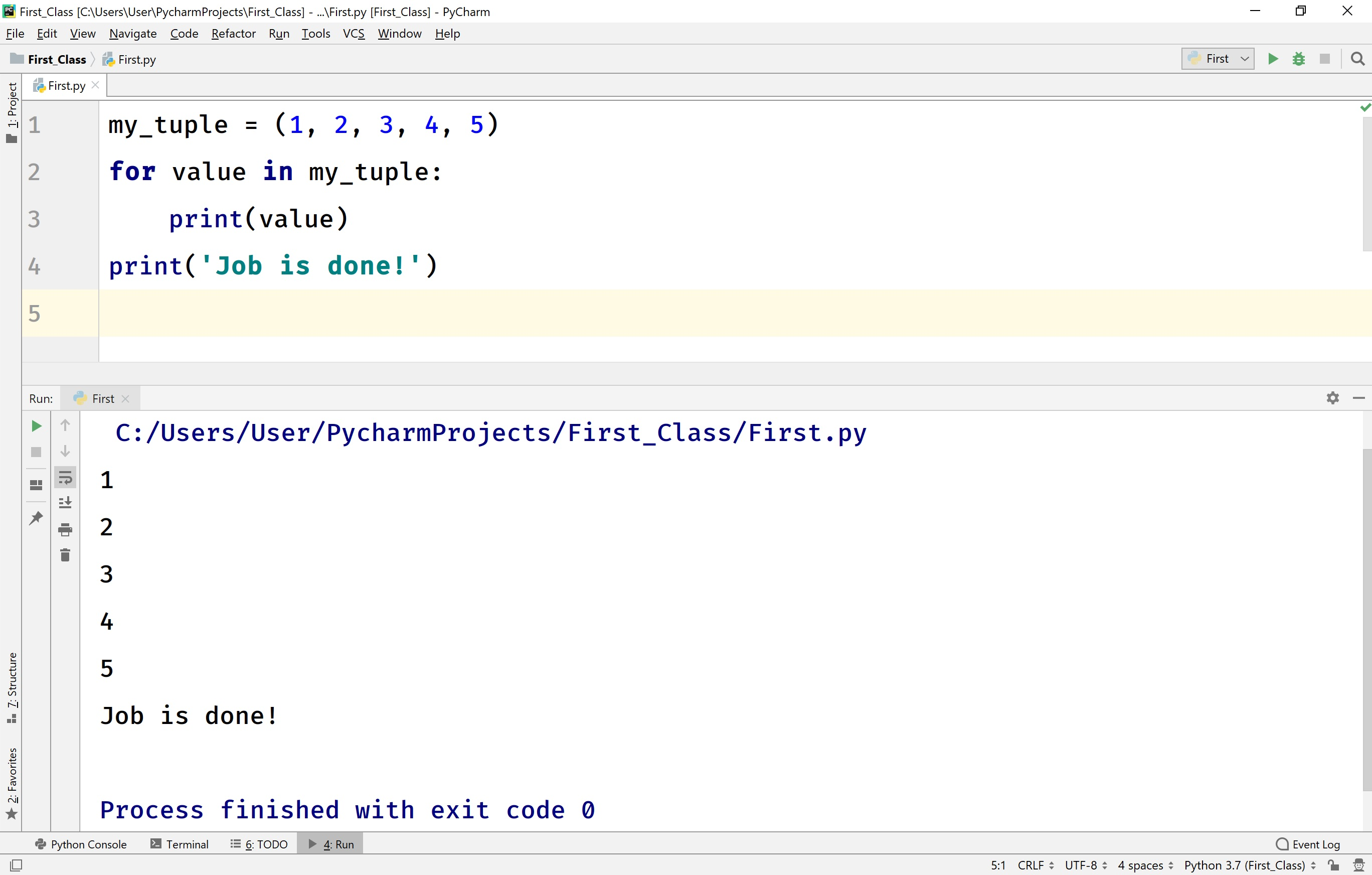# Python code to illustrate for statement with Set

``````
# Python code to illustrate for statement with Set
my_set = {1, 2, 3, 4, 5}
for value in my_set:
print(value)
print('Job is done!')
```
```

When we run the above code, it produces the output as follows.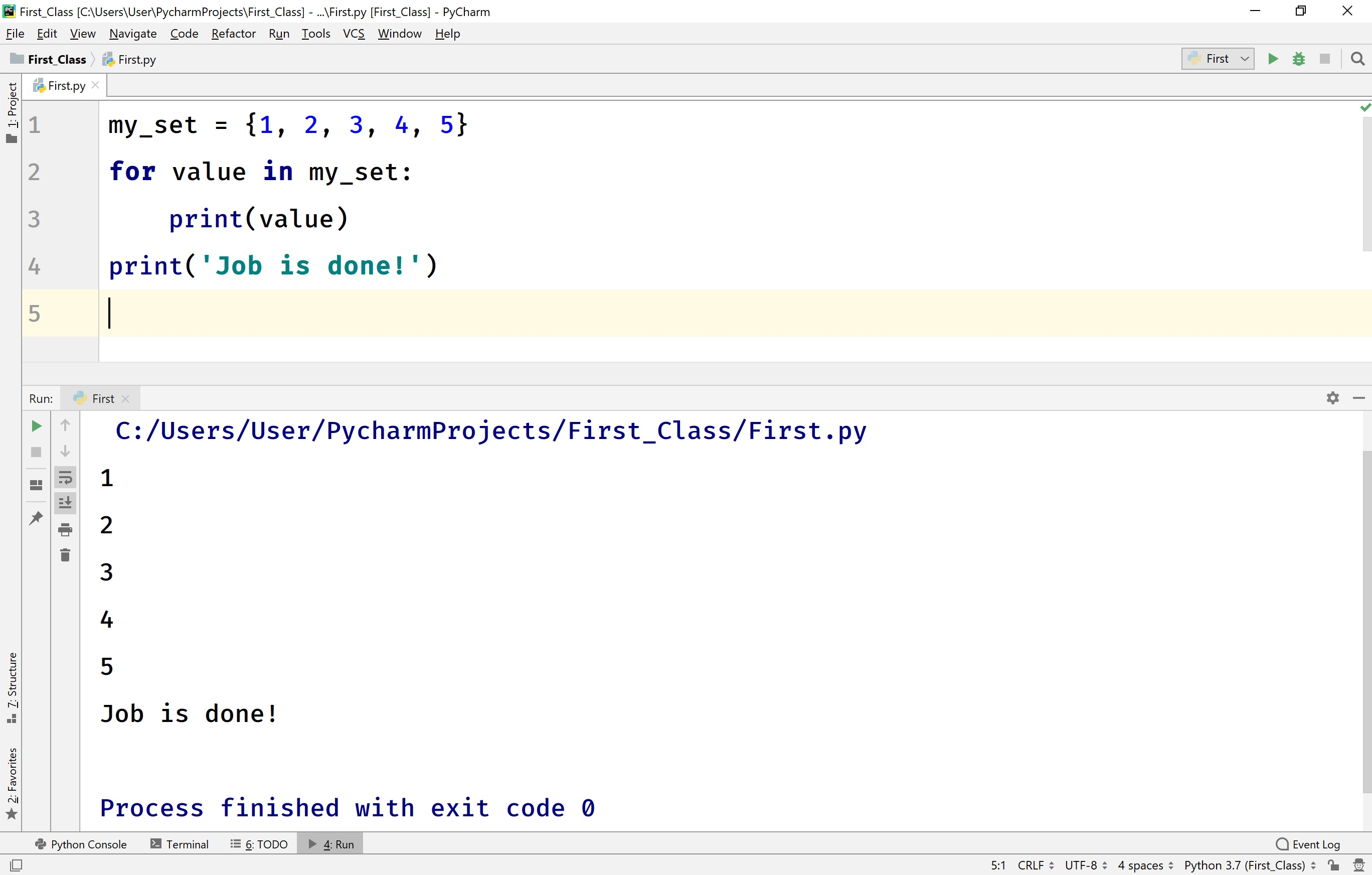# Python code to illustrate for statement with Dictionary

``````
# Python code to illustrate for statement with Dictionary
my_dictionary = {1:'Rama', 2:'Seetha', 3:'Heyaansh', 4:'Gouthami', 5:'Raja'}
for key, value in my_dictionary.items():
print(f'{key} --> {value}')
print('Job is done!')
```
```

When we run the above code, it produces the output as follows.# Python code to illustrate for statement with String

``````
# Python code to illustrate for statement with String
for item in 'Python':
print(item)
print('Job is done!')
```
```

When we run the above code, it produces the output as follows.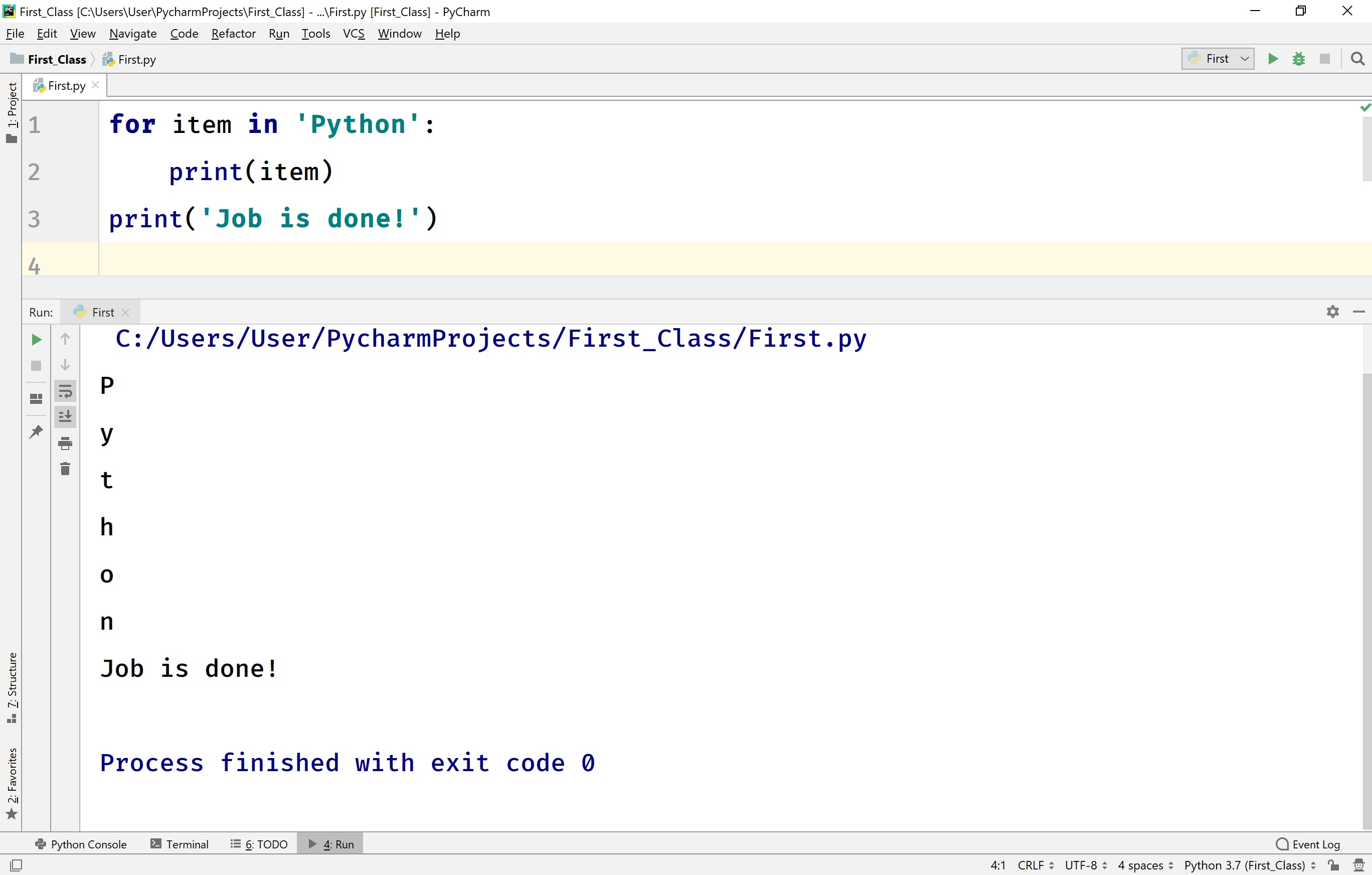# Python code to illustrate for statement with Range function

``````
# Python code to illustrate for statement with Range function
for value in range(1, 6):
print(value)
print('Job is done!')
```
```

When we run the above code, it produces the output as follows.## for statement with 'else' clause in Python

In Python, the else clause can be used with a for a statement. The else block is gets executed whenever the for statement is does not terminated with a break statement. But, if the for loop is terminated with break statement then else block doesn't execute.

# Python code to illustrate for statement with else clause

``````
# Here, else block is gets executed because break statement does not executed
for item in 'Python':
if item == 'x':
break
print(item)
else:
print('else block says for is successfully completed!')
print('Job is done!!')
```
```

When we run the above code, the else block is gets executed because a break statement does not execute. It produces the output as follows.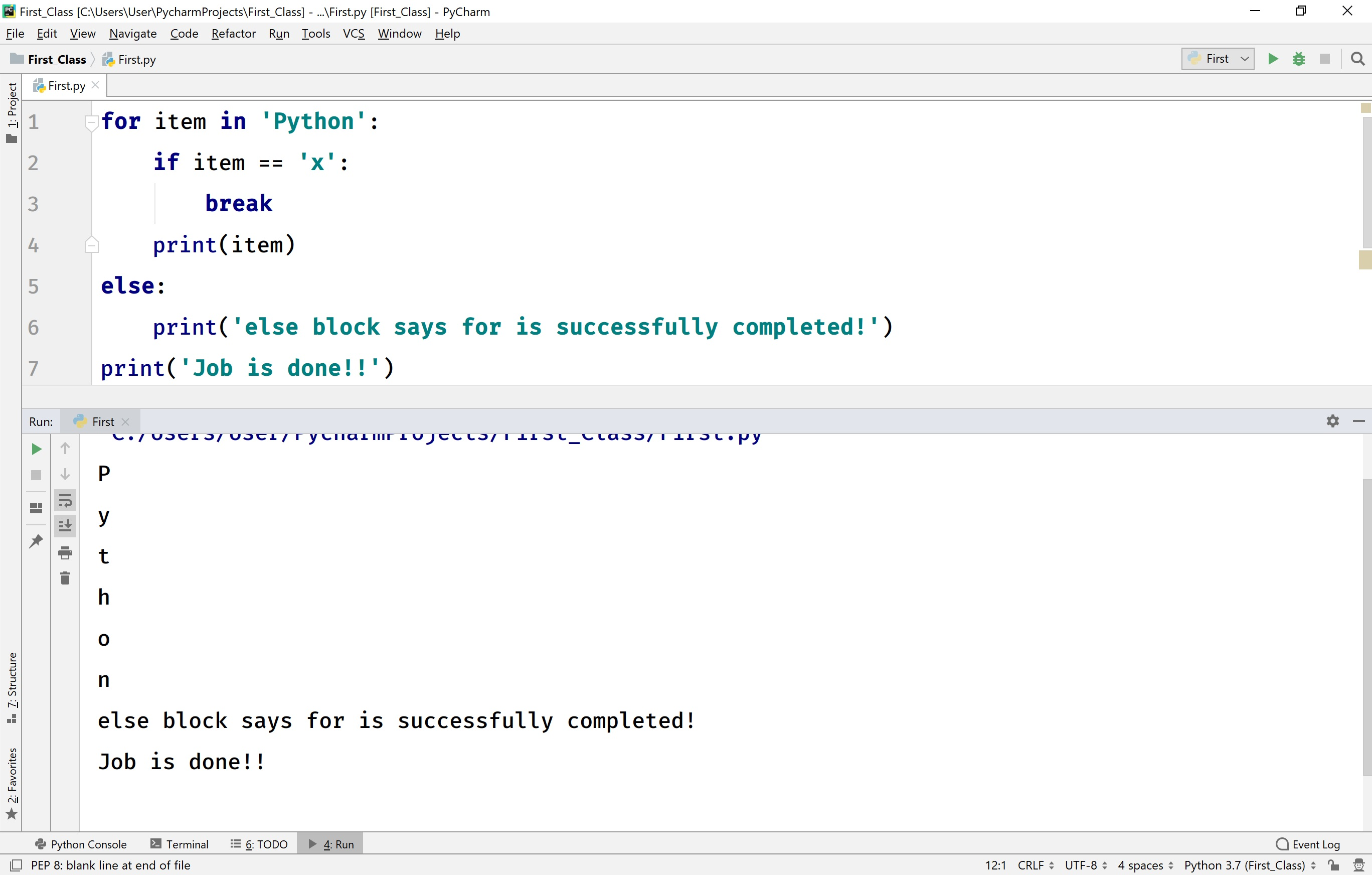# Python code to illustrate for statement with else clause

``````
# Here, else block does not gets executed because break statement terminates the loop.
for item in 'Python':
if item == 'y':
break
print(item)
else:
print('else block says for is successfully completed!')
print('Job is done!!')
```
```

When we run the above code, the else block does not get executed because a break statement terminates the loop. It produces the output as follows.